# Tree Data StructureThis post is part of the Data Structures series.

When you first learn to code, it’s common to learn arrays as the “main data structure.”

Eventually, you will learn about `hash tables` too. If you are pursuing a Computer Science degree, you have to take a class on data structure. You will also learn about `linked lists`, `queues`, and `stacks`. Those data structures are called “linear” data structures because they all have a logical start and a logical end.

When we start learning about `trees` and `graphs`, it can get really confusing. We don’t store data in a linear way. Both data structures store data in a specific way.

This post is to help you better understand the Tree Data Structure and to clarify any confusion you may have about it.

• What is a tree
• Examples of trees
• Its terminology and how it works
• How to implement tree structures in code.

Let’s start this learning journey. :)

## Definition

When starting out programming, it is common to understand better the linear data structures than data structures like trees and graphs.

Trees are well-known as a non-linear data structure. They don’t store data in a linear way. They organize data hierarchically.

## Let’s dive into real life examples!

What do I mean when I say in a hierarchical way?

Imagine a family tree with relationships from all generation: grandparents, parents, children, siblings, etc. We commonly organize family trees hierarchically.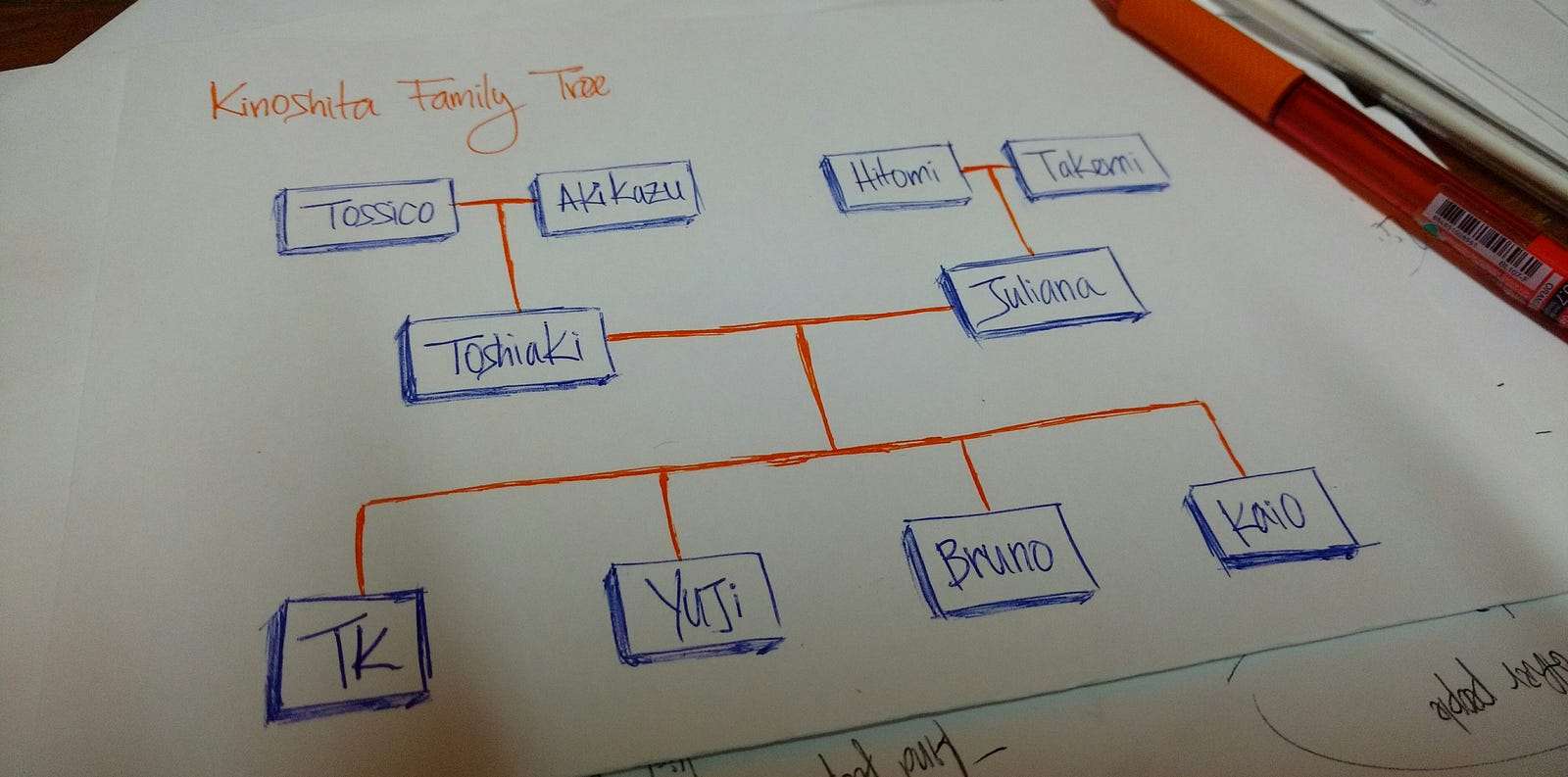The above drawing is is my family tree. `Tossico, Akikazu, Hitomi,` and `Takemi` are my grandparents.

`Toshiaki` and `Juliana`are my parents.

`TK, Yuji, Bruno`, and `Kaio` are the children of my parents (me and my brothers).

An organization’s structure is another example of a hierarchy.In HTML, the Document Object Model (DOM) works as a tree.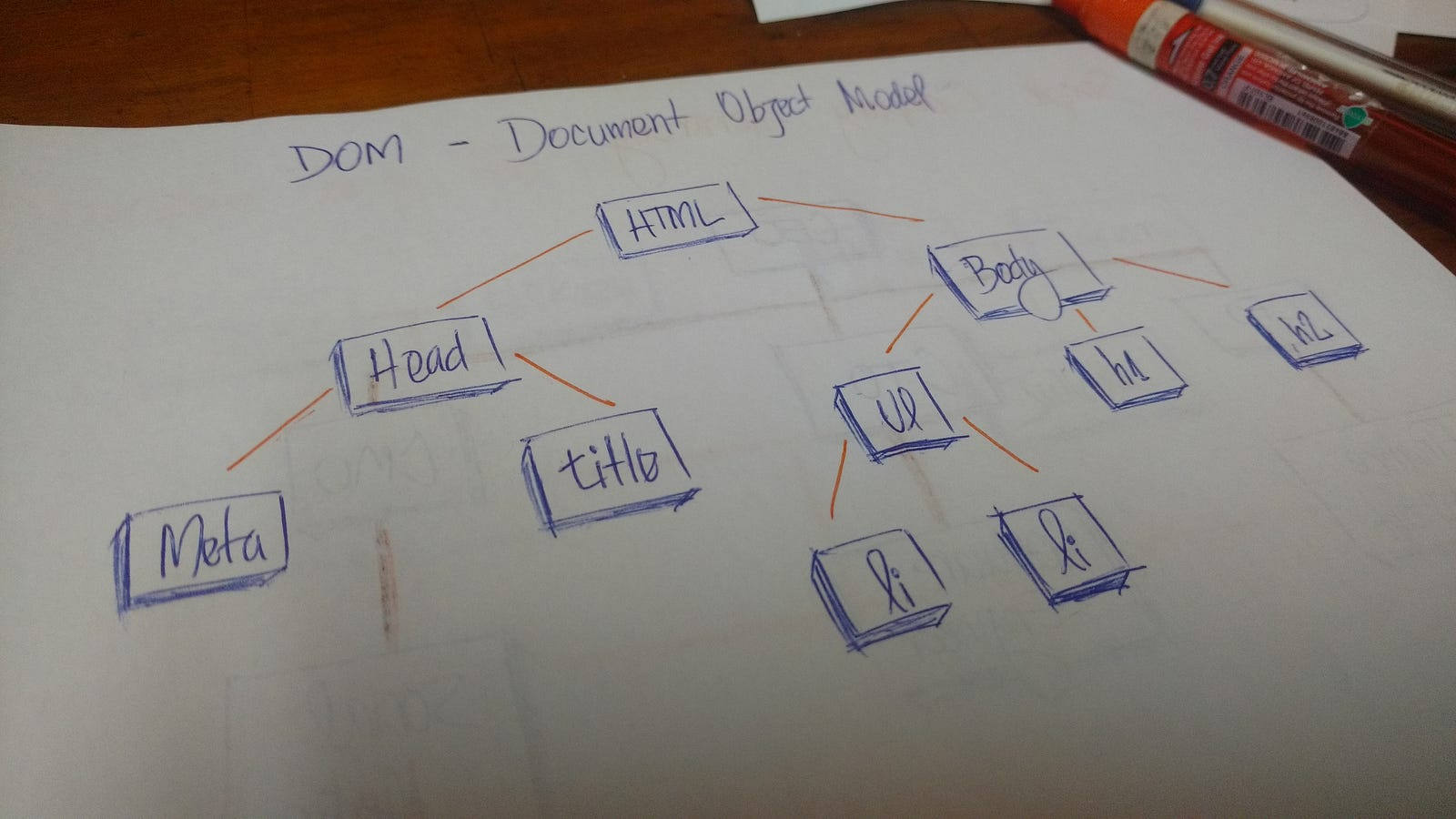The `HTML` tag contains other tags. We have a `head` tag and a `body` tag. Those tags contains specific elements. The `head` tag has `meta` and `title` tags. The `body` tag has elements that show in the user interface, for example, `h1`, `a`, `li`, etc.

## A technical definition

A `tree` is a collection of entities called `nodes`. Nodes are connected by `edges`. Each `node` contains a `value` or `data`, and it may or may not have a `child node` .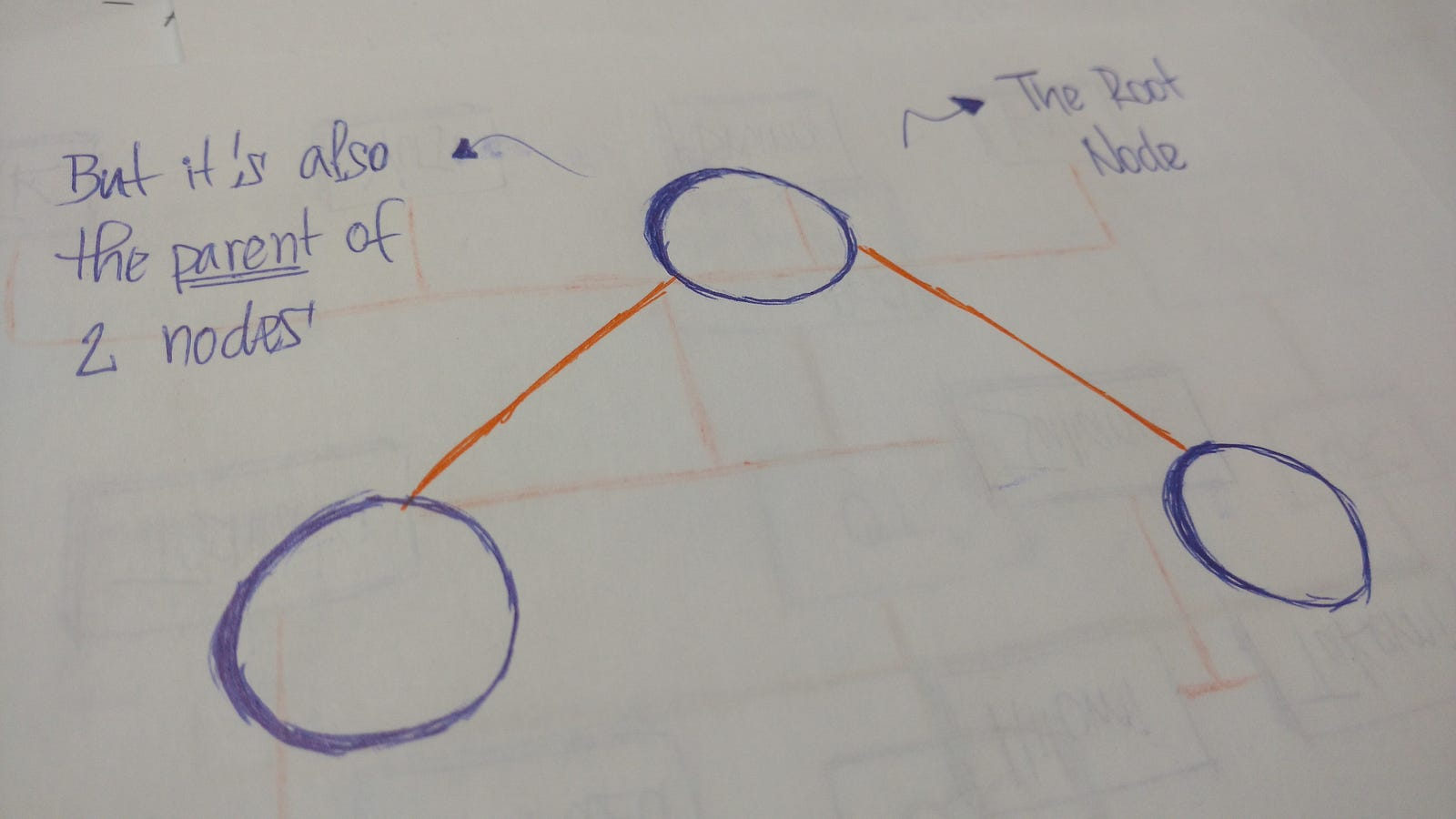The `first node` of the `tree` is called the `root`. If this `root node` is connected by another `node`, the `root` is then a `parent node` and the connected `node` is a `child`.All`Tree nodes` are connected by links called `edges`. It’s an important part of `trees`, because it’s manages the relationship between `nodes`.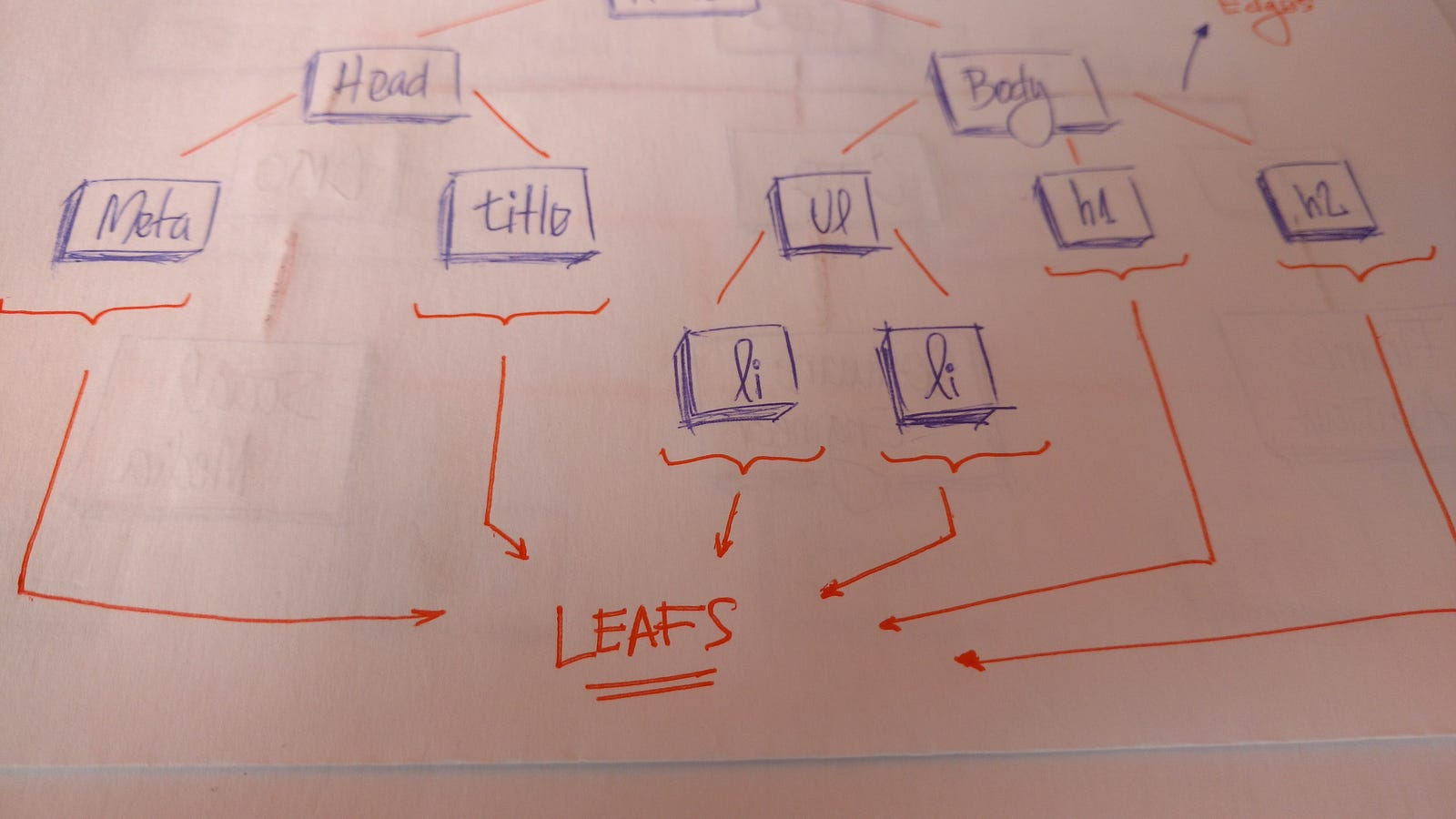`Leaves` are the last `nodes` on a `tree.` They are nodes without children. Like real trees, we have the `root`, `branches`, and finally the `leaves`.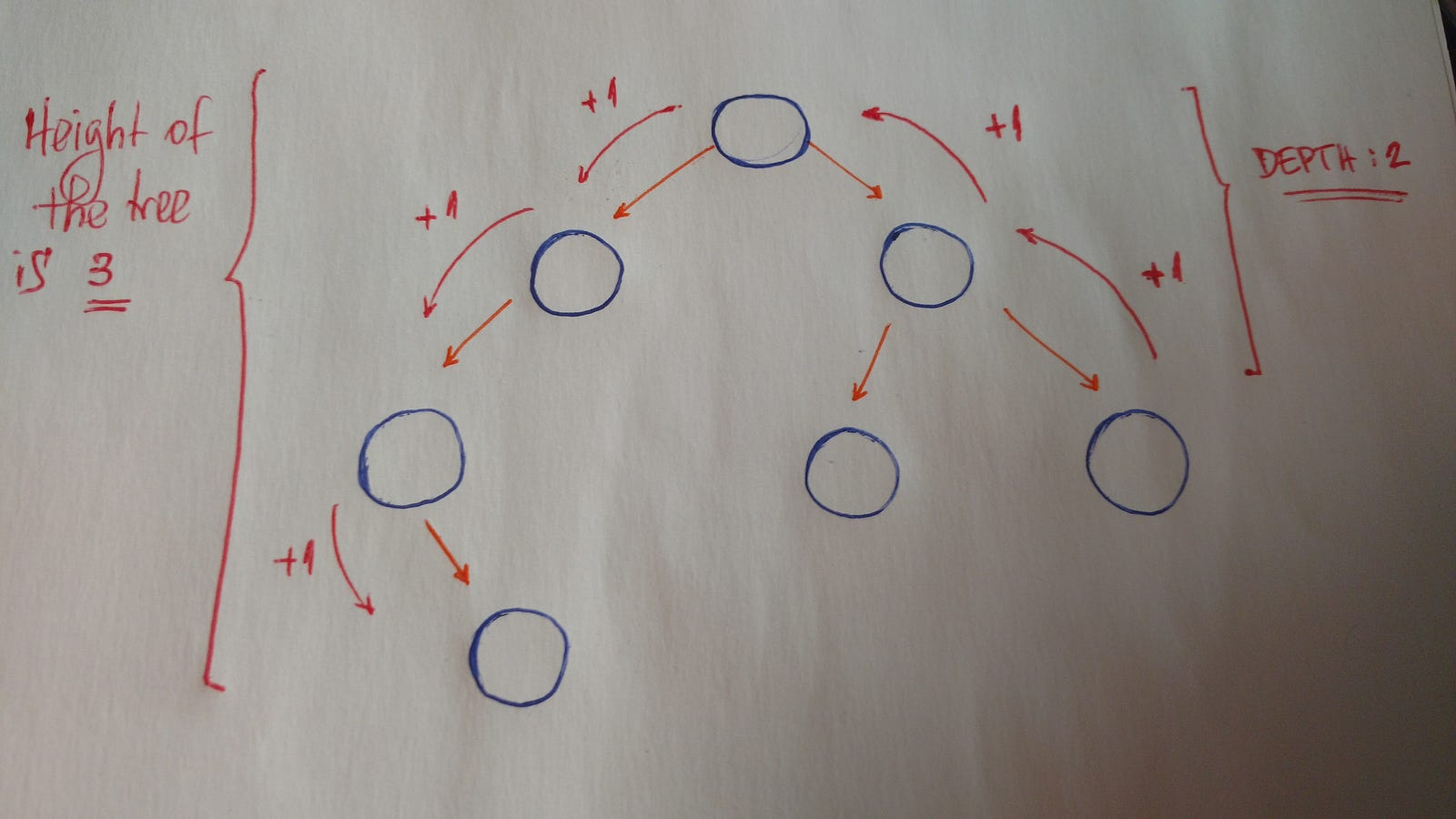Other important concepts to understand are `height` and `depth`.

The `height` of a `tree` is the length of the longest path to a `leaf`.

The `depth` of a `node` is the length of the path to its `root`.

## Terminology summary

• Root is the topmost `node` of the `tree`

• Edge is the link between two `nodes`

• Child is a `node` that has a `parent node`

• Parent is a `node` that has an `edge` to a `child node`

• Leaf is a `node` that does not have a `child node` in the `tree`

• Height is the length of the longest path to a `leaf`

• Depth is the length of the path to its `root`

## Binary trees

Now we will discuss a specific type of `tree`. We call it the `binary tree`.

“In computer science, a binary tree is a tree data structure in which each node has at the most two children, which are referred to as the left child and the right child.” — Wikipedia

So let’s look at an example of a `binary tree`.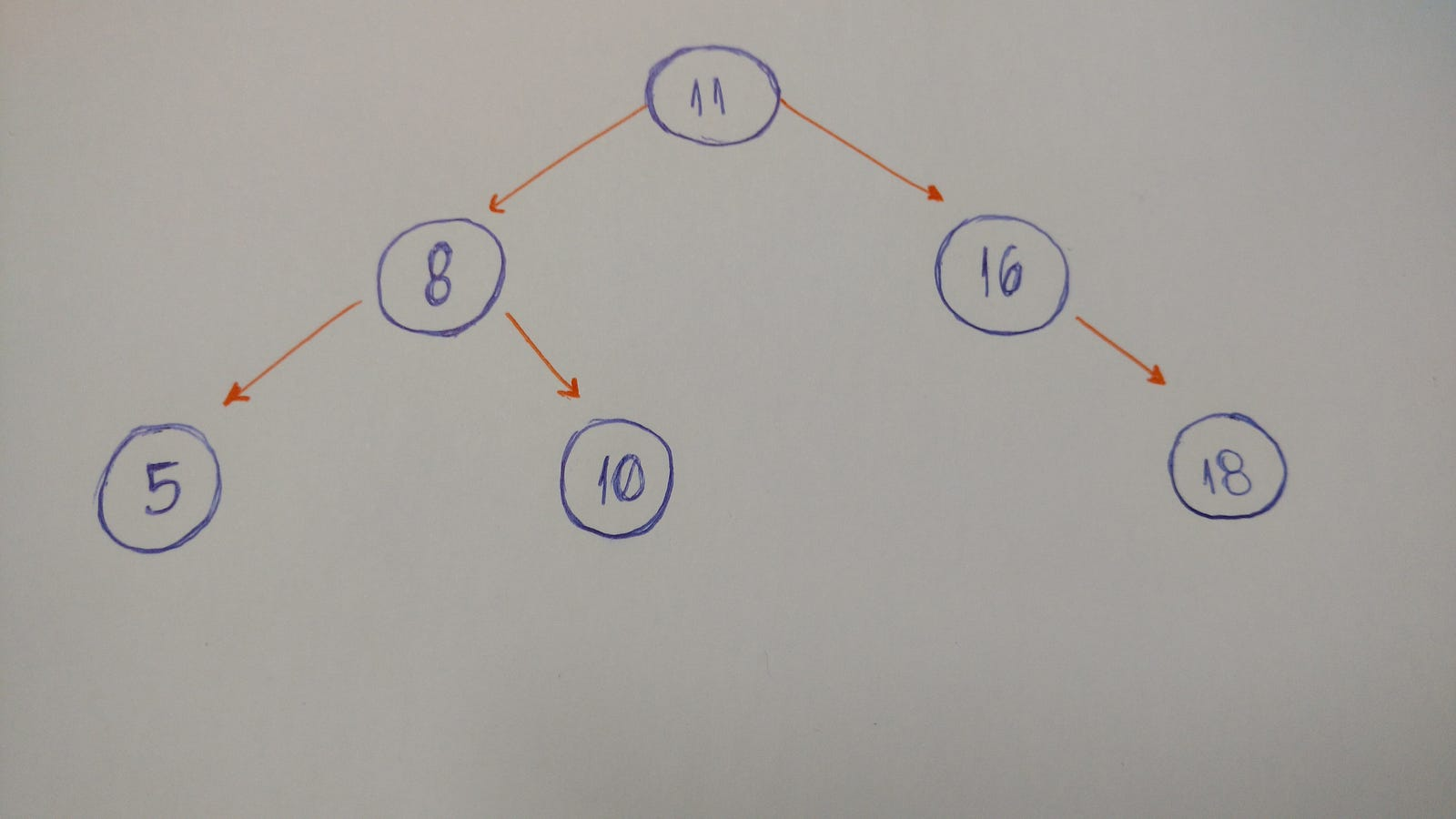## Let’s code a binary tree

The first thing we need to keep in mind when we implement a `binary tree` is that it is a collection of `nodes`. Each `node` has three attributes: `value`, `left_child`, and `right_child`.

How do we implement a simple `binary tree` that initializes with these three properties?

Let’s take a look.

``````class BinaryTree:
def __init__(self, value):
self.value = value
self.left_child = None
self.right_child = None
``````

Here it is. Our `binary tree` class.

When we instantiate an object, we pass the `value` (the data of the node) as a parameter. Look at the `left_child` and the `right_child`. Both are set to `None`.

Why?

Because when we create our `node`, it doesn’t have any children. We just have the `node data`.

Let’s test it:

``````tree = BinaryTree('a')
print(tree.value) # a
print(tree.left_child) # None
print(tree.right_child) # None
``````

That’s it.

We can pass the `string``a`’ as the `value` to our `Binary Tree node`. If we print the `value`, `left_child`, and `right_child`, we can see the values.

Let’s go to the insertion part. What do we need to do here?

We will implement a method to insert a new `node` to the `right` and to the `left`.

Here are the rules:

• If the current `node` doesn’t have a `left child`, we just create a new `node`and set it to the current node’s `left_child`.

• If it does have the `left child`, we create a new node and put it in the current `left child`’s place. Allocate this `left child node` to the new node’s `left child`.

Let’s draw it out. :)Here’s the code:

``````def insert_left(self, value):
if self.left_child == None:
self.left_child = BinaryTree(value)
else:
new_node = BinaryTree(value)
new_node.left_child = self.left_child
self.left_child = new_node
``````

Again, if the current node doesn’t have a `left child`, we just create a new node and set it to the current node’s `left_child`. Or else we create a new node and put it in the current `left child`’s place. Allocate this `left child node` to the new node’s `left child`.

And we do the same thing to insert a `right child node`.

``````def insert_right(self, value):
if self.right_child == None:
self.right_child = BinaryTree(value)
else:
new_node = BinaryTree(value)
new_node.right_child = self.right_child
self.right_child = new_node
``````

Done! :)

But not entirely. We still need to test it.

Let’s build the following `tree`: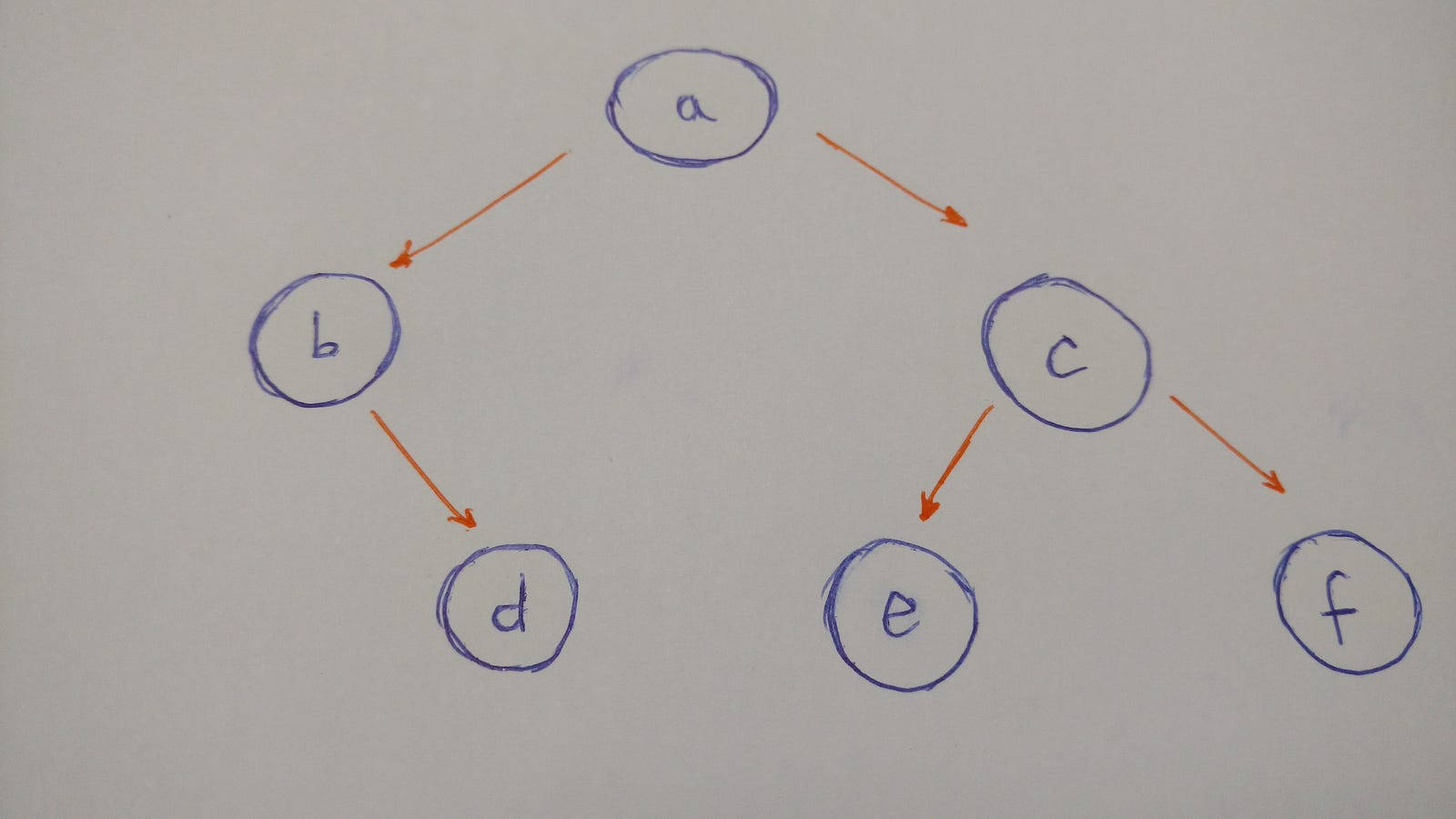To summarize the illustration of this tree:

• `a` `node` will be the `root` of our `binary Tree`

• `a` `left child` is `b` `node`

• `a` `right child` is `c` `node`

• `b` `right child` is `d` `node` (`b` `node` doesn’t have a `left child`)

• `c` `left child` is `e` `node`

• `c` `right child` is `f` `node`

• both `e` and `f` `nodes` do not have children

So here is the code for the `tree`:

``````a_node = BinaryTree('a')
a_node.insert_left('b')
a_node.insert_right('c')

b_node = a_node.left_child
b_node.insert_right('d')

c_node = a_node.right_child
c_node.insert_left('e')
c_node.insert_right('f')

d_node = b_node.right_child
e_node = c_node.left_child
f_node = c_node.right_child

print(a_node.value) # a
print(b_node.value) # b
print(c_node.value) # c
print(d_node.value) # d
print(e_node.value) # e
print(f_node.value) # f
``````

Insertion is done.

Now we have to think about `tree` traversal.

We have two options here: Depth-First Search (DFS) and Breadth-First Search (BFS).

So let’s dive into each tree traversal type.

### Depth-First Search (DFS)

DFS explores a path all the way to a leaf before backtracking and exploring another path. Let’s take a look at an example with this type of traversal.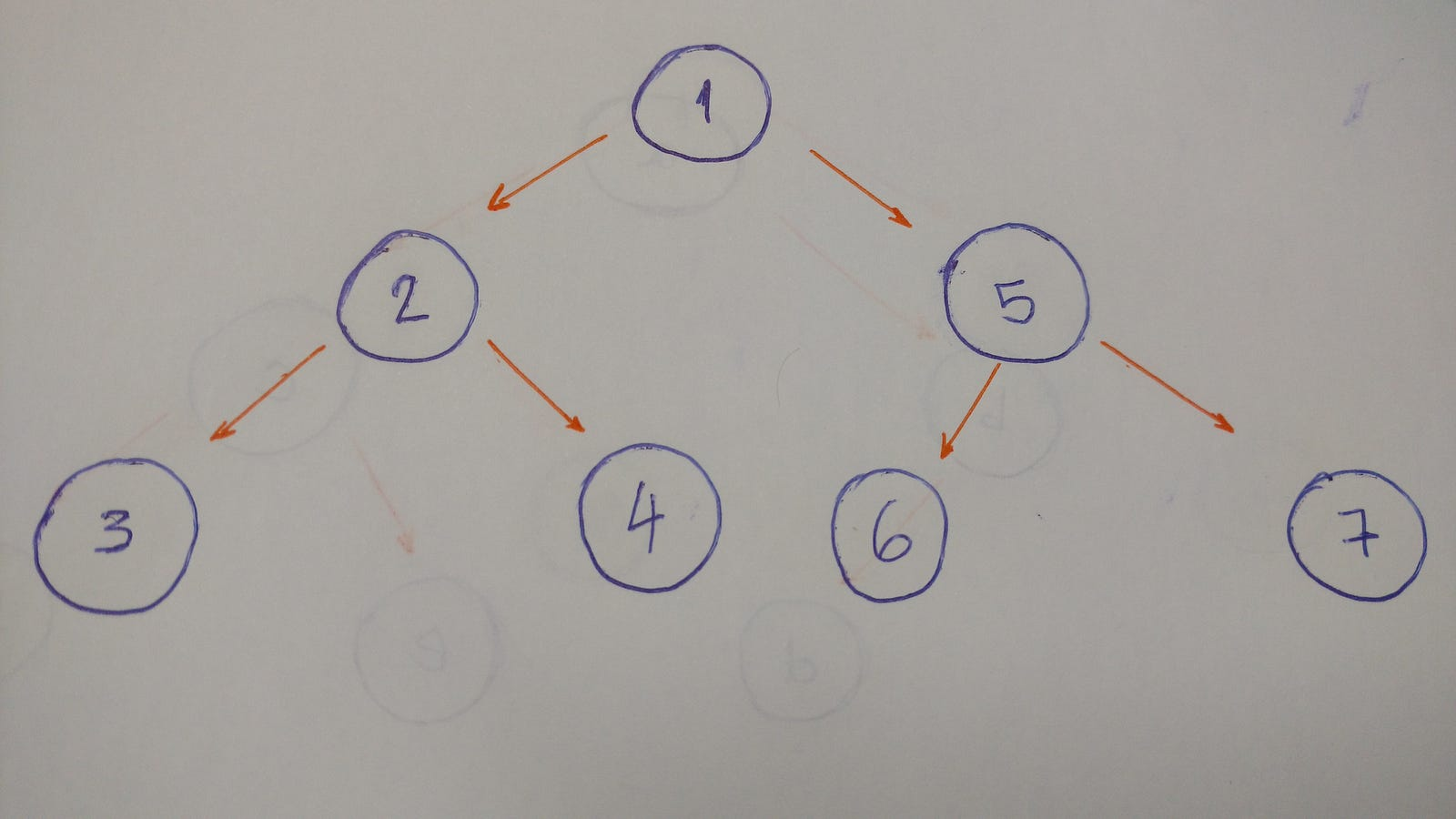The result for this algorithm will be 1–2–3–4–5–6–7.

Why?

Let’s break it down.

1. Print the root value.

2. Go to the `left child` (2). Print it.

3. Then go to the `left child` (3). Print it. (This `node` doesn’t have any children)

4. Backtrack and go the `right child` (4). Print it. (This `node` doesn’t have any children)

5. Backtrack to the `root` `node` and go to the `right child` (5). Print it.

6. Go to the `left child` (6). Print it. (This `node` doesn’t have any children)

7. Backtrack and go to the `right child` (7). Print it. (This `node` doesn’t have any children)

8. Done.

When we go deep to the leaf and backtrack, this is called DFS algorithm.

Now that we are familiar with this traversal algorithm, we will discuss the three types of DFS: `pre-order`, `in-order`, and `post-order`.

### Pre-order

This is exactly what we did in the above example.

``````def pre_order(self):
print(self.value)

if self.left_child:
self.left_child.pre_order()

if self.right_child:
self.right_child.pre_order()
``````

Print the current node, go to the left until it didn't have a left child, and then go to the right.

The result is exactly as the above algorithm: 1–2–3–4–5–6–7.

### In-orderThe result of the in-order algorithm for this `tree` example is 3–2–4–1–6–5–7.

The left first, the middle second, and the right last.

Now let’s code it.

``````def in_order(self):
if self.left_child:
self.left_child.in_order()

print(self.value)

if self.right_child:
self.right_child.in_order()
``````

### Post-orderThe result of the `post order` algorithm for this `tree` example is 3–4–2–6–7–5–1.

The left first, the right second, and the middle last.

Let’s code this.

``````def post_order(self):
if self.left_child:
self.left_child.post_order()

if self.right_child:
self.right_child.post_order()

print(self.value)
``````

These algorithms go deeply in the tree.

BFS algorithm traverses the `tree` level by level and depth by depth.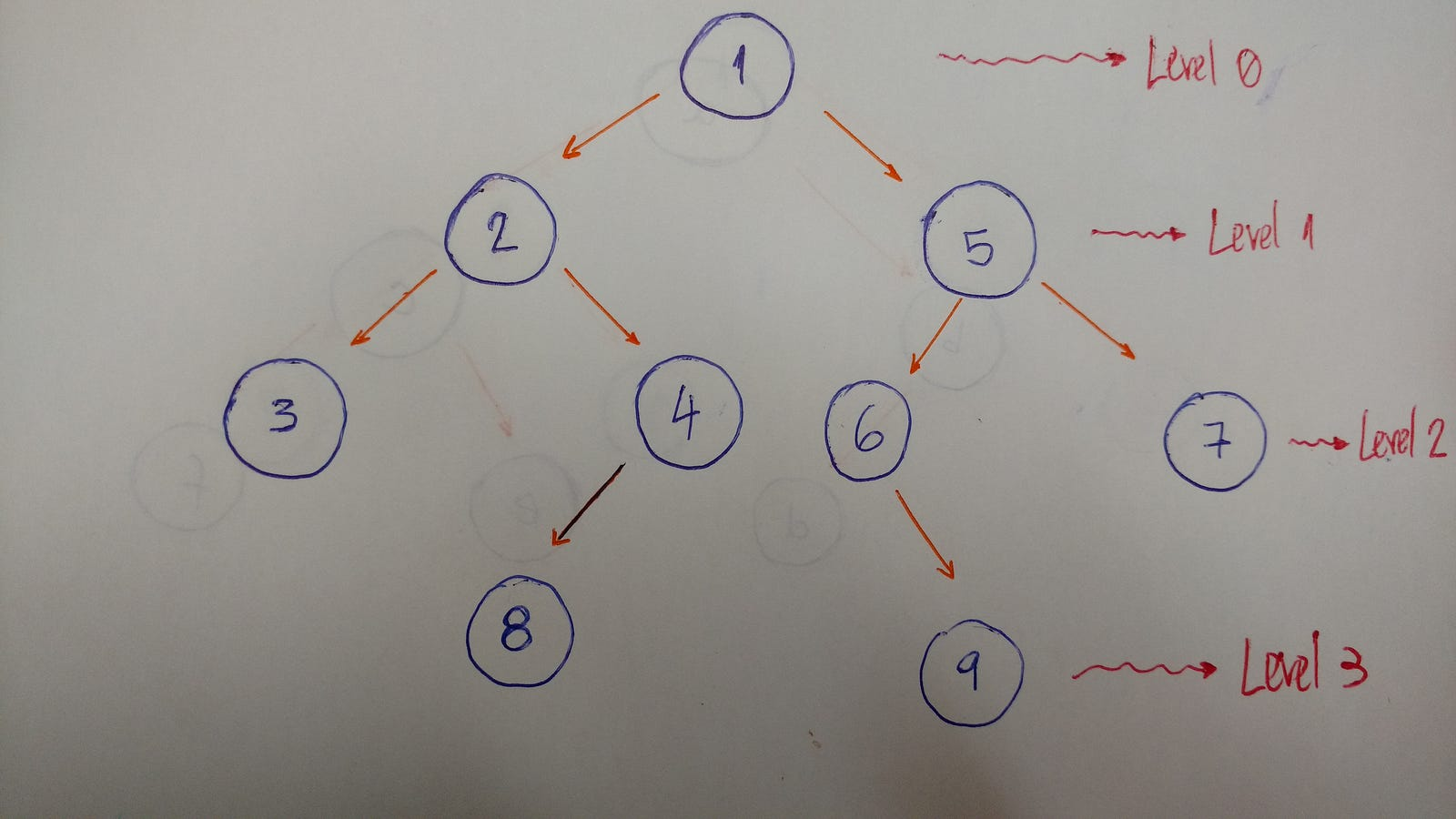The level 0 is only the root node. Level 1 is for children. Level 2 grandchildren. And so on.

Here is an example that helps to better explain this algorithm:So we traverse level by level. In this example, the result is 1–2–5–3–4–6–7. Why?

• Level/Depth 0: only `node` with value 1

• Level/Depth 1: `nodes` with values 2 and 5

• Level/Depth 2: `nodes` with values 3, 4, 6, and 7

Now let’s code it.

``````def bfs(self):
queue = Queue()
queue.put(self)

while not queue.empty():
current_node = queue.get()
print(current_node.value)

if current_node.left_child:
queue.put(current_node.left_child)

if current_node.right_child:
queue.put(current_node.right_child)
``````

To implement a BFS algorithm, we use the `queue` data structure to help.

How does it work?

Here’s the explanation.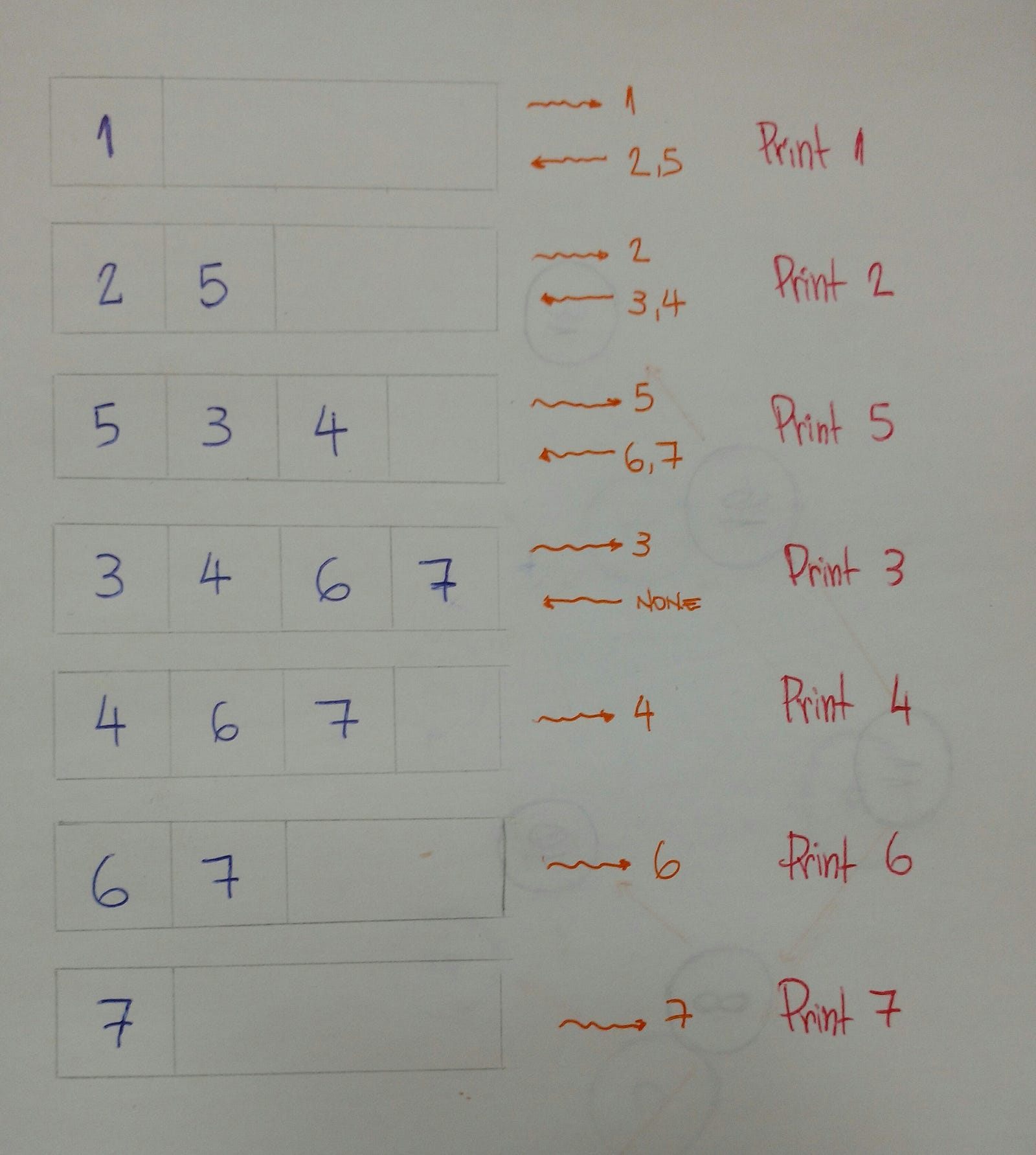• Add the root node to the queue

• We run the algorithm until the queue doesn't anything

• Get the first element in queue

• Print its value

• Add its children in the queue (if it has)

• Run this algorithm again if we have any node in the queue

The illustration: we get 1 first (root node), print its value, and add its children to the queue: left first, right last. Our queue has nodes inside it, so run it again. Get the first node in the queue (2) and print it. Add its children. And we do this algorithm for each node in the queue.

As we use the queue to add and get elements, we pop when get the next node.

## Binary Search tree

“A Binary Search Tree is sometimes called ordered or sorted binary trees, and it keeps its values in sorted order, so that lookup and other operations can use the principle of binary search” — Wikipedia

An important property of a `Binary Search Tree` is that the value of a `Binary Search Tree` `node` is larger than the value of the offspring of its `left child`, but smaller than the value of the offspring of its `right child.`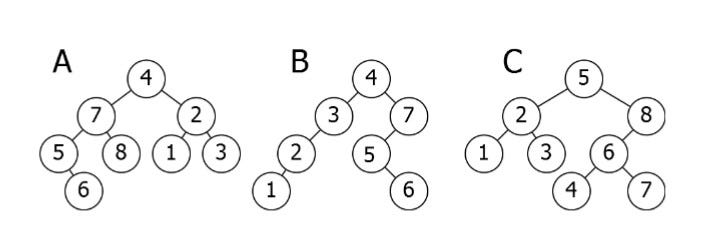Here is a breakdown of the above illustration:

• A is inverted. The `subtree` 7–5–8–6 needs to be on the right side, and the `subtree` 2–1–3 needs to be on the left.

• B is the only correct option. It satisfies the `Binary Search Tree` property.

• C has one problem: the `node` with the value 4. It needs to be on the left side of the `root` because it is smaller than 5.

## Let’s code a Binary Search Tree!

Now it’s time to code!

What will we see here? We will insert new nodes, search for a value, delete nodes, and the balance of the `tree`.

Let’s start.

### Insertion: adding new nodes to our tree

Imagine that we have an empty `tree` and we want to add new `nodes` with the following values in this order: 50, 76, 21, 4, 32, 100, 64, 52.

The first thing we need to know is if 50 is the `root` of our tree.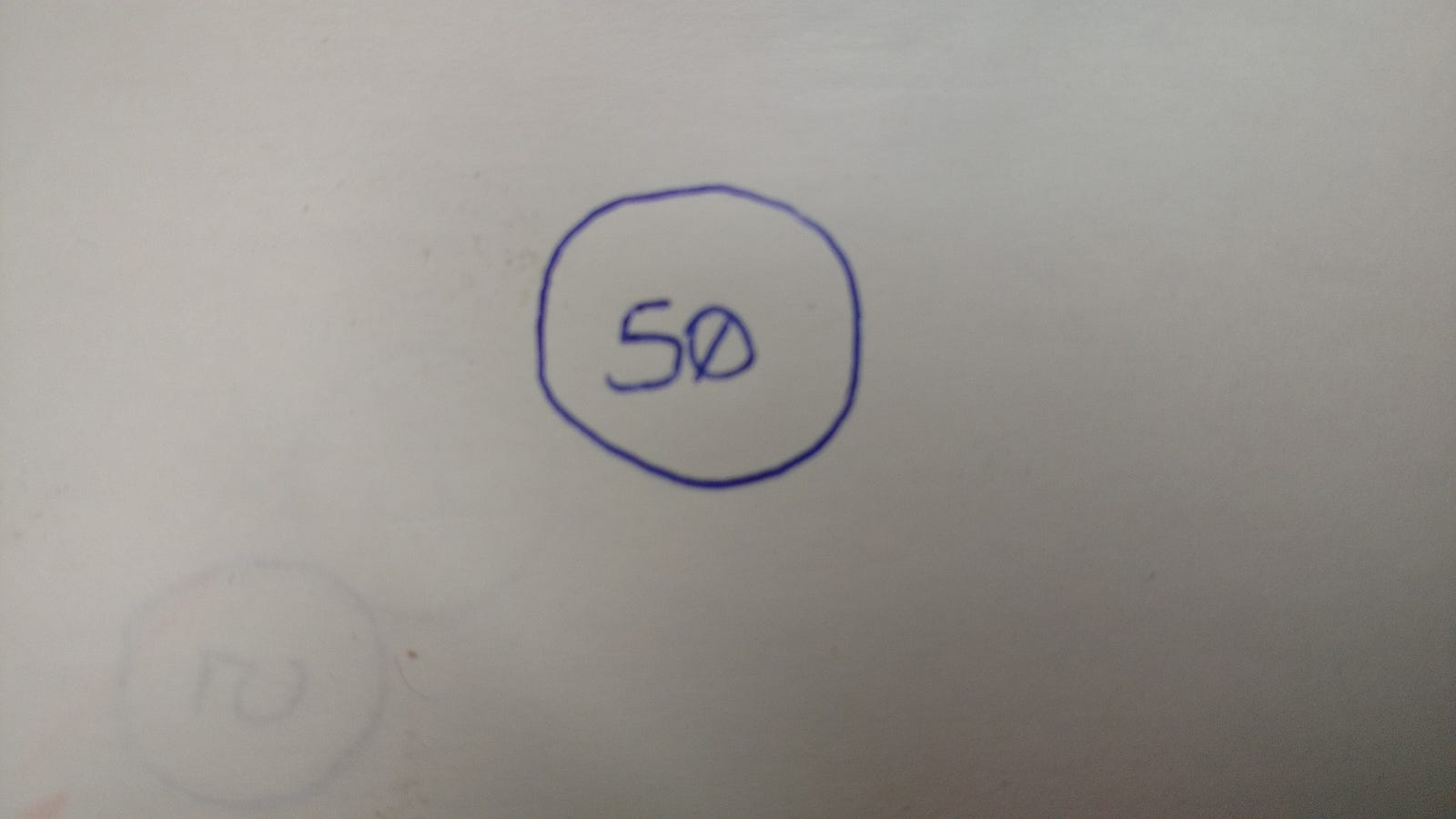We can now start inserting `node` by `node`.

• 76 is greater than 50, so insert 76 on the right side.

• 21 is smaller than 50, so insert 21 on the left side.

• 4 is smaller than 50. `Node` with value 50 has a `left child` 21. Since 4 is smaller than 21, insert it on the left side of this `node`.

• 32 is smaller than 50. `Node` with value 50 has a `left child` 21. Since 32 is greater than 21, insert 32 on the right side of this `node`.

• 100 is greater than 50. `Node` with value 50 has a `right child` 76. Since 100 is greater than 76, insert 100 on the right side of this `node`.

• 64 is greater than 50. `Node` with value 50 has a `right child`76. Since 64 is smaller than 76, insert 64 on the left side of this `node`.

• 52 is greater than 50. `Node` with value 50 has a `right child`76. Since 52 is smaller than 76, `node` with value 76 has a `left child` 64. 52 is smaller than 64, so insert 54 on the left side of this `node`.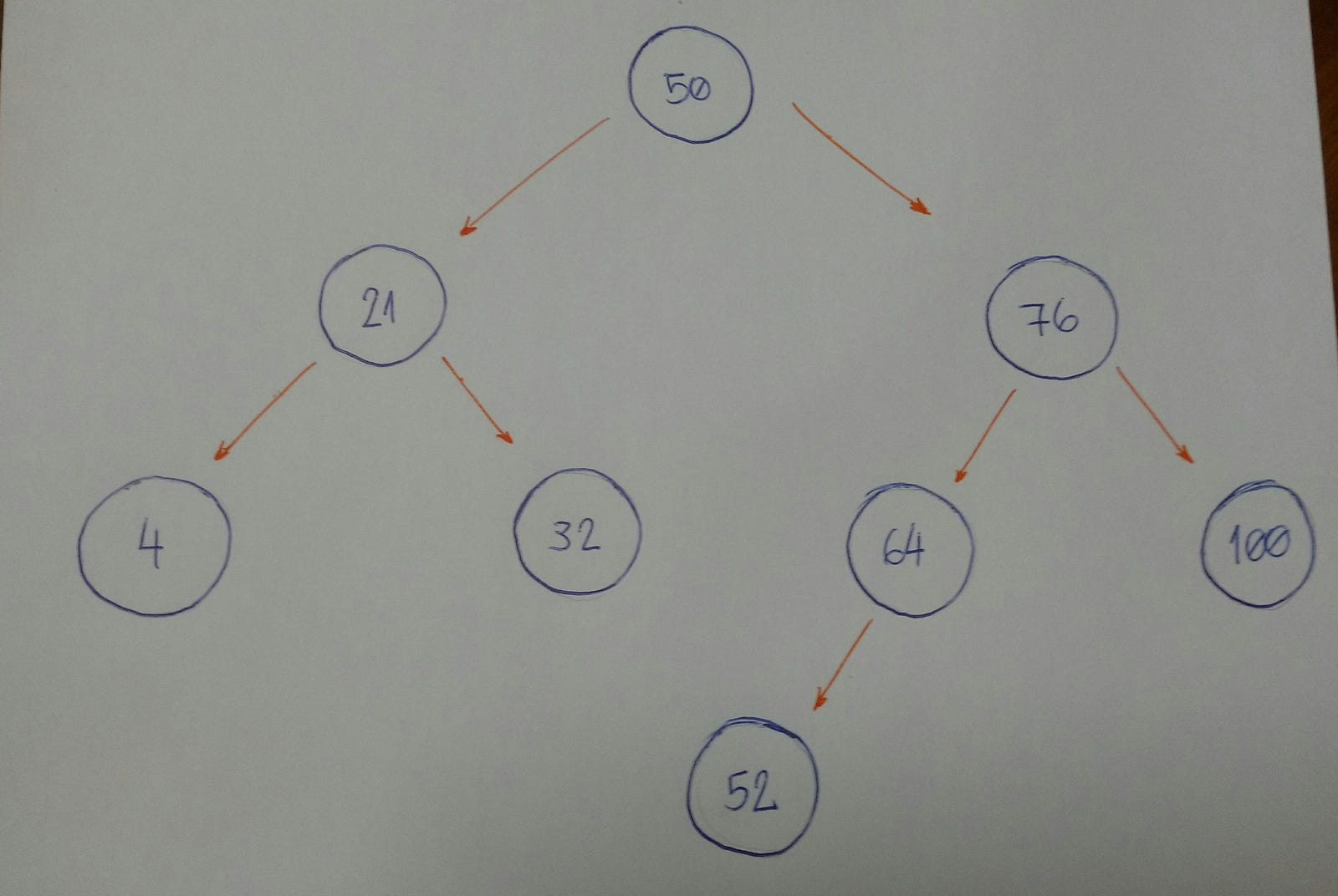Do you notice a pattern here?

Let’s break it down.

Now let’s code it.

``````class BinarySearchTree:
def __init__(self, value):
self.value = value
self.left_child = None
self.right_child = None

def insert_node(self, value):
if value <= self.value and self.left_child:
self.left_child.insert_node(value)
elif value <= self.value:
self.left_child = BinarySearchTree(value)
elif value > self.value and self.right_child:
self.right_child.insert_node(value)
else:
self.right_child = BinarySearchTree(value)
``````

It seems very simple.

The powerful part of this algorithm is the recursion part, which is on line 9 and line 13. Both lines of code call the `insert_node` method, and use it for its `left` and `right` `children`, respectively. Lines `11` and `15` are the ones that do the insertion for each `child`.

### Let’s search for the node value... Or not...

The algorithm that we will build now is about doing searches. For a given value (integer number), we will say if our `Binary Search Tree` does or does not have that value.

An important item to note is how we defined the tree insertion algorithm. First we have our `root` `node`. All the left `subtree` `nodes` will have smaller values than the `root` `node`. And all the right `subtree` `nodes` will have values greater than the `root` `node`.

Let’s take a look at an example.

Imagine that we have this `tree`.Now we want to know if we have a node based on value 52.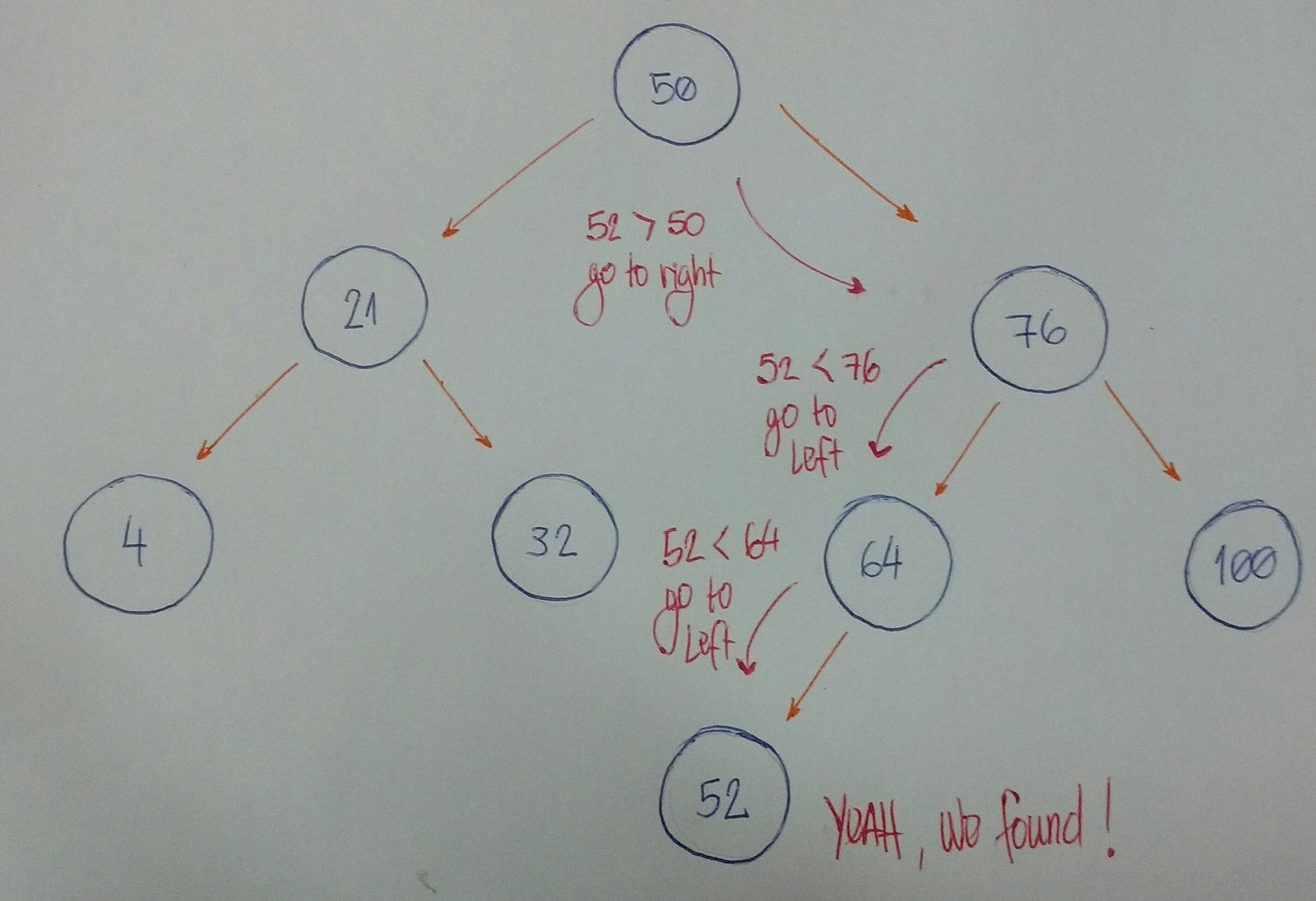Let’s break it down.

Now let’s code it.

``````class BinarySearchTree:
def __init__(self, value):
self.value = value
self.left_child = None
self.right_child = None

def find_node(self, value):
if value < self.value and self.left_child:
return self.left_child.find_node(value)
if value > self.value and self.right_child:
return self.right_child.find_node(value)

return value == self.value
``````

Let’s break down the code:

• Lines 8 and 9 fall under rule #1.

• Lines 10 and 11 fall under rule #2.

• Line 13 falls under rule #3.

How do we test it?

Let’s create our `Binary Search Tree` by initializing the `root` `node` with the value 15.

``````bst = BinarySearchTree(15)
``````

And now we will insert many new `nodes`.

``````bst.insert_node(10)
bst.insert_node(8)
bst.insert_node(12)
bst.insert_node(20)
bst.insert_node(17)
bst.insert_node(25)
bst.insert_node(19)
``````

For each inserted `node`, we will test if our `find_node` method really works.

``````print(bst.find_node(15)) # True
print(bst.find_node(10)) # True
print(bst.find_node(8)) # True
print(bst.find_node(12)) # True
print(bst.find_node(20)) # True
print(bst.find_node(17)) # True
print(bst.find_node(25)) # True
print(bst.find_node(19)) # True
``````

Yeah, it works for these given values! Let’s test for a value that doesn’t exist in our `Binary Search Tree`.

``````print(bst.find_node(0)) # False
``````

Oh yeah.

Our search is done.

### Deletion: removing and organizing

Deletion is a more complex algorithm because we need to handle different cases. For a given value, we need to remove the `node` with this value. Imagine the following scenarios for this `node` : it has no `children`, has a single `child`, or has two `children`.

• Scenario #1: A `node` with no `children` (`leaf` `node`).
``````#        |50|                              |50|
#      /      \                           /    \
#    |30|     |70|   (DELETE 20) --->   |30|   |70|
#   /    \                                \
# |20|   |40|                             |40|
``````

If the `node` we want to delete has no children, we simply delete it. The algorithm doesn’t need to reorganize the `tree`.

• Scenario #2: A `node` with just one child (`left` or `right` child).
``````#        |50|                              |50|
#      /      \                           /    \
#    |30|     |70|   (DELETE 30) --->   |20|   |70|
#   /
# |20|
``````

In this case, our algorithm needs to make the parent of the `node` point to the `child` node. If the `node` is the `left child`, we make the parent of the `left child` point to the `child`. If the `node` is the `right child` of its parent, we make the parent of the `right child` point to the `child`.

• Scenario #3: A `node` with two children.
``````#        |50|                              |50|
#      /      \                           /    \
#    |30|     |70|   (DELETE 30) --->   |40|   |70|
#   /    \                             /
# |20|   |40|                        |20|
``````

When the `node` has 2 children, we need to find the `node` with the minimum value, starting from the `node`’s`right child`. We will put this `node` with minimum value in the place of the `node` we want to remove.

It’s time to code.

``````def remove_node(self, value, parent):
if value < self.value and self.left_child:
return self.left_child.remove_node(value, self)
elif value < self.value:
return False
elif value > self.value and self.right_child:
return self.right_child.remove_node(value, self)
elif value > self.value:
return False
else:
if self.left_child is None and self.right_child is None and self == parent.left_child:
parent.left_child = None
self.clear_node()
elif self.left_child is None and self.right_child is None and self == parent.right_child:
parent.right_child = None
self.clear_node()
elif self.left_child and self.right_child is None and self == parent.left_child:
parent.left_child = self.left_child
self.clear_node()
elif self.left_child and self.right_child is None and self == parent.right_child:
parent.right_child = self.left_child
self.clear_node()
elif self.right_child and self.left_child is None and self == parent.left_child:
parent.left_child = self.right_child
self.clear_node()
elif self.right_child and self.left_child is None and self == parent.right_child:
parent.right_child = self.right_child
self.clear_node()
else:
self.value = self.right_child.find_minimum_value()
self.right_child.remove_node(self.value, self)

return True
``````
• To use the `clear_node` method: set the `None` value to all three attributes — (`value`, `left_child`, and `right_child`)
``````def clear_node(self):
self.value = None
self.left_child = None
self.right_child = None
``````
• To use the `find_minimum_value` method: go way down to the left. If we can’t find anymore nodes, we found the smallest one.
``````def find_minimum_value(self):
if self.left_child:
return self.left_child.find_minimum_value()
else:
return self.value
``````

Now let’s test it.

We will use this `tree` to test our `remove_node` algorithm.

``````#        |15|
#      /      \
#    |10|     |20|
#   /    \    /    \
# |8|   |12| |17| |25|
#              \
#              |19|
``````

Let’s remove the `node` with the `value` 8. It’s a `node` with no child.

``````print(bst.remove_node(8, None)) # True
bst.pre_order_traversal()

#     |15|
#   /      \
# |10|     |20|
#    \    /    \
#   |12| |17| |25|
#          \
#          |19|
``````

Now let’s remove the `node` with the `value` 17. It’s a `node` with just one child.

``````print(bst.remove_node(17, None)) # True
bst.pre_order_traversal()

#        |15|
#      /      \
#    |10|     |20|
#       \    /    \
#      |12| |19| |25|
``````

Finally, we will remove a `node` with two children. This is the `root` of our `tree`.

``````print(bst.remove_node(15, None)) # True
bst.pre_order_traversal()

#        |19|
#      /      \
#    |10|     |20|
#        \        \
#        |12|     |25|
``````

The tests are now done. :)

## That’s all for now!

We learned a lot here.

Congrats on finishing this dense content. It’s really tough to understand a concept that we do not know. But you did it ☺

Have fun, keep learning and coding.

Here are my Medium, Twitter & GitHub accounts ☺